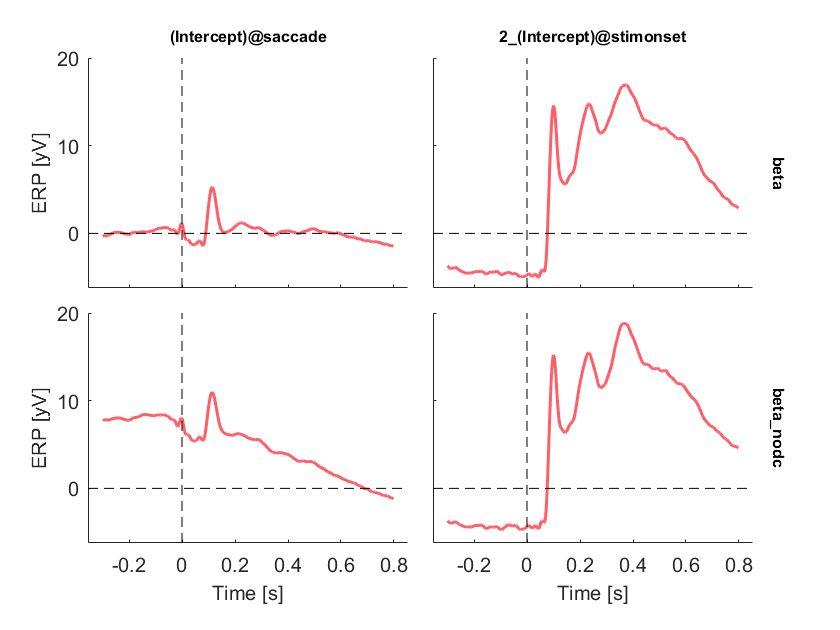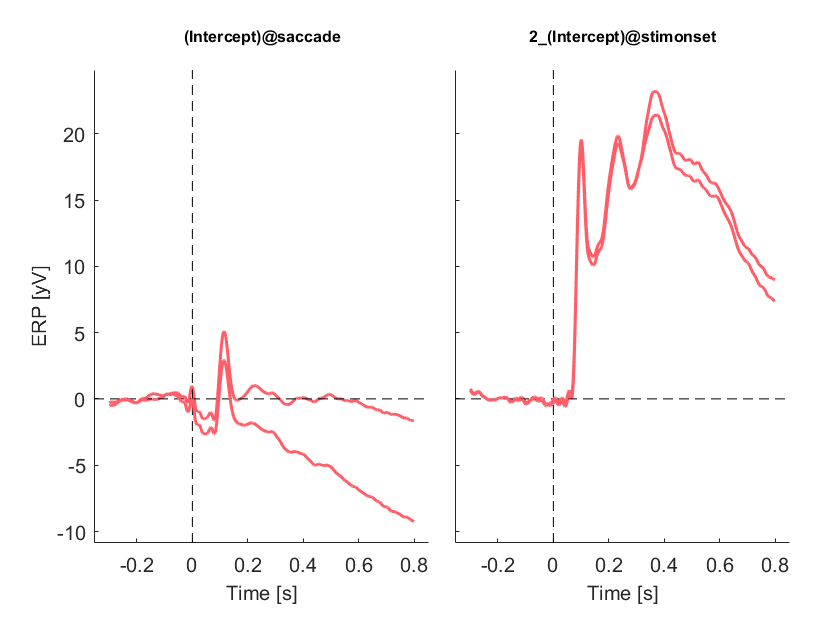init_unfold
Starting unfold toolbox. Adding subfolders and other toolboxes to path... Done.

# Introduction

Subjects are exploring a face stimulus. Using an eyetracker and the EEG-Eye toolbox we add markers of saccade onsets into our EEG-Data. The data were cleaned for eye-movement related artefacts using ICA.
In this tutorial we try to disentangle stimulus related ERPs and microsaccade related ERPs.
Find the data at: https://osf.io/wbz7x/
EEG = struct with fields:
setname: 'face_saccades_opendata' filename: 'face_saccades_opendata_fig10.set' filepath: 'C:\Users\behinger\Desktop' subject: '' group: '' condition: '' session: [] comments: 'Data to re-create Figure 10 in Ehinger & Dimigen, 2018, BioRxiv. Please do not use this data for other purposes without asking first (olaf.dimigen@hu-berlin.de)' nbchan: 40 trials: 1 pnts: 2070402 srate: 500 xmin: 0 xmax: 4.1408e+03 times: [1×2070402 double] data: [40×2070402 single] icaact: [] icawinv: [] icasphere: [] icaweights: [] icachansind: [] chanlocs: [1×40 struct] urchanlocs: [] chaninfo: [1×1 struct] ref: 'averef' event: [1×7661 struct] urevent: [1×7661 struct] eventdescription: {'' '' '' '' '' '' '' '' '' '' '' '' '' ''} epoch: [] epochdescription: {} reject: [1×1 struct] stats: [1×1 struct] specdata: [] specicaact: [] splinefile: '' icasplinefile: '' dipfit: [] history: '↵EEG.setname='MSAFF_01';↵EEG = eeg_checkset( EEG );↵EEG = eeg_checkset( EEG );↵EEG.etc.eeglabvers = '14.1.2'; % this tracks which version of EEGLAB is being used, you may ignore it↵EEG = pop_loadset('filename','face_saccades_opendata.set','filepath','M:\\Dropbox\\shared folders_main\\deconv\\figures\\toolbox_paper\\redo_figure10_to_match_opendata\\');↵EEG = eeg_checkset( EEG );↵pop_eegplot( EEG, 1, 1, 1);↵EEG = pop_select( EEG,'nochannel',{'L-Dia-X-(px)' 'L-Dia-Y-(px)' 'R-Dia-X-(px)' 'R-Dia-Y-(px)'});↵EEG = eeg_checkset( EEG );↵EEG = pop_saveset( EEG, 'filename','face_saccades_opendata_fig10.set','filepath','M:\\Dropbox\\shared folders_main\\deconv\\figures\\toolbox_paper\\redo_figure10_to_match_opendata\\');↵EEG = eeg_checkset( EEG );↵EEG = pop_select( EEG,'nochannel',{'L-GAZE-X-(px)' 'L-GAZE-Y-(px)' 'R-GAZE-X-(px)' 'R-GAZE-Y-(px)'});↵EEG = eeg_checkset( EEG );↵EEG.etc.eeglabvers = '14.1.1'; % this tracks which version of EEGLAB is being used, you may ignore it' saved: 'justloaded' etc: [1×1 struct] datfile: []
EEG = eeg_checkset(EEG);

# Defining the design

cfgDesign = [];
% We use intercept-only formulas because we are only interested in the overlap for now
cfgDesign.formula = {'y ~ 1','y~1'};
EEG = uf_designmat(EEG,cfgDesign);
Multiple events with separate model-formula detected Modeling 2871 event(s) of [saccade] using formula: y~1 Modeling 479 event(s) of [stimonset] using formula: y~1

# Timeshift

In order to compensate for the inevitable linear overlap between ERPs, we want to use timeshifting / prepare the designmatrix for the deconvolution step. In this step we generate for each column of the designmatrix timeshifted (by t = 1, t=2, t=3 ...) and append them to the designmatrix.
cfgTimeexpand = [];
cfgTimeexpand.timelimits = [-.3,0.8];
EEG = uf_timeexpandDesignmat(EEG,cfgTimeexpand);
uf_timeexpandDesignmat(): Timeexpanding the designmatrix... ...done

# Cleaning of data

% A simple threshold function taken from ERPLAB
winrej = uf_continuousArtifactDetect(EEG,'amplitudeThreshold',250);
WARNING: boundary events were not found. So, basicrap.m will be applied over the full range of data. Please wait. This might take several seconds... 58 segments were marked. A total of 133034 samples (6.43 percent of data) was marked as bad.
% We remove very noisy data segments (>250mV) from the designmatrix
EEG = uf_continuousArtifactExclude(EEG,struct('winrej',winrej));
Portions of data removed split up by each event in EEG.event Type: S 99 modelled eventtime: 1054.90s rejected eventtime: 16.33s percent removed: 1.5% Type: S100 modelled eventtime: 1.10s rejected eventtime: 1.10s percent removed: 100.0% Type: S200 modelled eventtime: 1.10s rejected eventtime: 0.00s percent removed: 0.0% Type: buttonpress modelled eventtime: 526.90s rejected eventtime: 7.25s percent removed: 1.4% Type: fixation modelled eventtime: 862.05s rejected eventtime: 11.27s percent removed: 1.3% Type: saccade modelled eventtime: 861.57s rejected eventtime: 11.27s percent removed: 1.3% Type: stimonset modelled eventtime: 526.90s rejected eventtime: 8.34s percent removed: 1.6% Removing 6.43% of rows from design matrix (filling them with zeros)

# Fit the deconvolution model

EEG= uf_glmfit(EEG,'channel',);
uf_glmfit(): Fitting deconvolution model...solving the equation system solving electrode 32 (of 1 electrodes in total)... 12 iterations, took 0.2s LMfit finished

# Fit the non-deconvolution model

While epoching changes the EEG.data, the previous EEG.deconv fields & fits do not. Thus the toolbox allows to have two fits, a "dc beta" and a "no-dc beta" concurrently
% We need to provide the same winrej as before, so that approximately the same data is used for the model.
% Only approximately because in epoched data we need to remove a whole trial, whereas deconvolution allows
% partial trial-data to be used.
% Be aware that if you decide to overwrite the EEG structure you need to reload your data to rerun your analysis on continuous data
EEG = uf_epoch(EEG,struct('winrej',winrej,'timelimits',cfgTimeexpand.timelimits));
Deleting 174 events due to data cleaning pop_epoch():3272 epochs selected Epoching... pop_epoch():3272 epochs generated eeg_checkset: found empty values for field 'timeSinceLastStimulus' filling with values of other events in the same epochs Event resorted by increasing latencies. pop_epoch(): checking epochs for data discontinuity Recalculating the EEG_epoch field using eeg_checkset, might take some time Event resorted by increasing latencies.
EEG = uf_glmfit_nodc(EEG);

# Plot the results

We choose a single electrode and plot all effects.
ufresult= uf_condense(EEG);
display(ufresult)
ufresult = struct with fields:
unfold: [1×1 struct] beta: [40×550×2 double] times: [1×550 double] chanlocs: [1×40 struct] param: [1×2 struct] beta_nodc: [40×550×2 double]
uf_plotParam(ufresult,'channel',32);
plotting all parametersIn order to directly compare the dc and non-dc model fits we can plot them in the same plot. Not the large baseline offset (no baseline correction so far) that is completly absent in the deconvoluted fit (this is an observeration we commonly have in many different datasets). For the comparison we do want to have baseline correction.
%first plot the deconvoluted betas
g = uf_plotParam(ufresult,'channel',32,'deconv',1,'baseline',[ufresult.times(1) 0]);
plotting all parametersperforming baseline correction
% now plot the non-deconvoluted betas
g = uf_plotParam(ufresult,'channel',32,'deconv',0,'baseline',[ufresult.times(1) 0],'gramm',g);
plotting all parametersperforming baseline correction New X or Y of different size given, all data from first gramm clearedIt is clear that disregarding the overlap leads to vastly different results for late components, but obviously not for early components, as no overlap happened here.